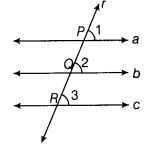# Prove that, lines which are parallel to the same line, are parallel to each other

Prove that, lines which are parallel to the same line, are parallel to each other.

Given Three lines a, b and c such that a||c and b||c.
To prove a||b
Construction Draw a transversal r cutting a, b and c at points P, Q and R, respectively.Proof Since, parallel lines a and c are intersected by the transversal r at points P and R, respectively.
∴∠1 = ∠3 [corresponding angles axiom] …(i)
Again, parallel lines b and c are intersected by the transversal r at points Q and R , respectively.
∴ ∠2 = ∠3 [corresponding angles axiom] …(ii)
From Eqs. (i) and (ii), we get ∠1 = ∠2
But these are corresponding angles.
∴ a||b [by converse of corresponding angles axiom]